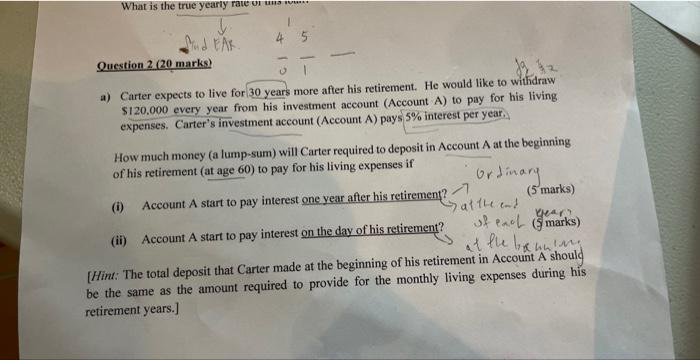# Question but why a(i) the nis not 29 Question $$2(20$$ marks) a) Carter expects to live for 30 years more after his retirement. He would like to withdraw $$\ 120.000$$ every year from his investment account (Account A) to pay for his living expenses. Carter's investment account (Account A) pays $$5 \%$$ interest per year. How much money (a lump-sum) will Carter required to deposit in Account $$A$$ at the beginning of his retirement (at age 60) to pay for his living expenses if (i) Account A start to pay interest one year after his retirement? $$\rightarrow$$ aft c 15 marks) (ii) Account A start to pay interest on the day of his retirement? of eac ( marks) [Hint: The total deposit that Carter made at the beginning of his retirement in Account A should be the same as the amount required to provide for the monthly living expenses during his retirement years.]Transcribed Image Text: Question $$2(20$$ marks) a) Carter expects to live for 30 years more after his retirement. He would like to withdraw $$\ 120.000$$ every year from his investment account (Account A) to pay for his living expenses. Carter's investment account (Account A) pays $$5 \%$$ interest per year. How much money (a lump-sum) will Carter required to deposit in Account $$A$$ at the beginning of his retirement (at age 60) to pay for his living expenses if (i) Account A start to pay interest one year after his retirement? $$\rightarrow$$ aft c 15 marks) (ii) Account A start to pay interest on the day of his retirement? of eac ( marks) [Hint: The total deposit that Carter made at the beginning of his retirement in Account A should be the same as the amount required to provide for the monthly living expenses during his retirement years.]
Transcribed Image Text: Question $$2(20$$ marks) a) Carter expects to live for 30 years more after his retirement. He would like to withdraw $$\ 120.000$$ every year from his investment account (Account A) to pay for his living expenses. Carter's investment account (Account A) pays $$5 \%$$ interest per year. How much money (a lump-sum) will Carter required to deposit in Account $$A$$ at the beginning of his retirement (at age 60) to pay for his living expenses if (i) Account A start to pay interest one year after his retirement? $$\rightarrow$$ aft c 15 marks) (ii) Account A start to pay interest on the day of his retirement? of eac ( marks) [Hint: The total deposit that Carter made at the beginning of his retirement in Account A should be the same as the amount required to provide for the monthly living expenses during his retirement years.]&#12304;General guidance&#12305;The answer provided below has been developed in a clear step by step manner.Step1/2(i) To calculate the lump sum amount that Carter will require at the beginning of his retirement if Account A starts to pay interest one year after his retirement, we can use the formula for present value of an annuity:$$\mathrm{{P}{V}={A}\times{\left[\frac{{{1}-{\left({1}+{r}\right)}^{{-{{n}}}}}}{{r}}\right]}}$$where PV is the present value, A is the annual withdrawal amount, r is the interest rate per year, and n is the number of years of withdrawals.In this case, A = $120,000, r = 0.05, and n = 30. However, since Account A starts to pay interest one year after his retirement, we need to adjust n to 29.PV = $$\mathrm{\{120},{000}\times{\left[\frac{{{1}-{\left({1}+{0.05}\right)}^{{-{{29}}}}}}{{0.05}}\right]}}$$PV =$1,939,341.95Therefore, Carter will require a lump sum amount of \$1,939,341.95 to deposit in Account A at ... See the full answer